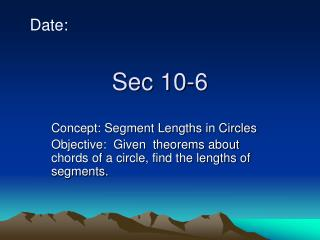DownloadDownload PresentationSec 10-6

# Sec 10-6

Download Presentation## Sec 10-6

- - - - - - - - - - - - - - - - - - - - - - - - - - - E N D - - - - - - - - - - - - - - - - - - - - - - - - - - -
##### Presentation Transcript

1. Date: Sec 10-6 Concept: Segment Lengths in Circles Objective: Given theorems about chords of a circle, find the lengths of segments.

2. B C A E D Thm 10.15: If 2 chords intersect in the interior of a circle, then the product of the lengths of the segments of one chord is equal to the product of the lengths of the segments of the other chord. EA·EB=EC·ED

3. B D x 9 C 12 18 A Example: Chords AB and CD intersect inside the circle. Find the value of x. 12·9=x·18 108=18x 6=x

4. 7 x 4 16 Example: Chords AB and CD intersect inside the circle. Find the value of x. x·4=7·16 4x=112 X=28

5. B A D E C Thm 10.16: If two secant segments share the same endpoint outside a circle, then the product of the lengths of one secant segment and the length of its external segment equals the product of the length of the other secant segment and the length of its external segment EA·EB=EC·ED

6. 11 10 12 x Example: Find the value of x. 11·21=12(12+x) 231=144+12x 87=12x 7.25=x

7. 29 x 35 15 Find the value of x x·(x+29)=15·50 X2+29x=750 X2+29x-750=0 Solve by quad. formula

8. A D E C Thm 10.17: If a secant segment and a tangent segment share an endpoint outside a circle, then the product of the length of the secant segment and the length of its external segment equals the square of the length of the tangent segment (EA)2=EC·ED

9. x 12 10 Example: find the value of x X2=10·22 X2=220 X=14.8

10. 6 5 x Example: find the value of x 62=x·(x+5) 36=x2+5x X2+5x-36=0 (x+9)(x-4)=0 x+9=0 x-4=0 X=-9 x=4

11. Today’s Work